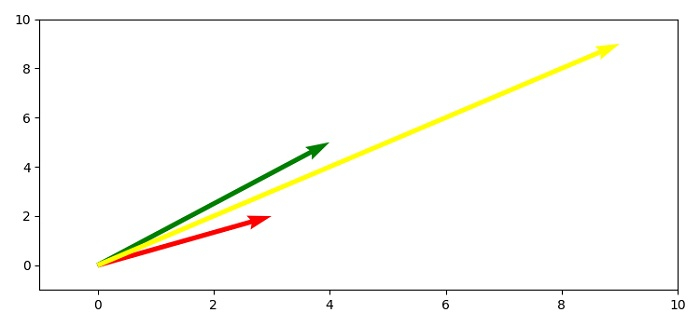# How does Python's Matplotlib.pyplot.quiver exactly work?

To work with quiver, we can take the following steps −

• Set the figure size and adjust the padding between and around the subplots.

• Create vector cordinates using numpy array.

• Get x, y, u and v data points.

• Create a new figure or activate an existing figure using figure() method.

• Get the current axis using gca() method.

• Set x and y limit of the axes.

• To redraw the current figure, use draw() method.

• To display the figure, use show() method.

## Example

import numpy as np
import matplotlib.pyplot as plt

plt.rcParams["figure.figsize"] = [7.50, 3.50]
plt.rcParams["figure.autolayout"] = True

soa = np.array([[0, 0, 3, 2], [0, 0, 4, 5], [0, 0, 9, 9]])
X, Y, U, V = zip(*soa)
plt.figure()

ax = plt.gca()
ax.quiver(X, Y, U, V, angles='xy', scale_units='xy', scale=1, color=['red', 'green', 'yellow'])
ax.set_xlim([-1, 10])
ax.set_ylim([-1, 10])

plt.draw()

plt.show()

## Output